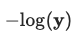## Negative Log-LikelihoodNegative log-likelihood is a loss function used in multi-class classification.

Calculated as $-log(\textbf{y})$, where $\textbf{y}$ is a prediction corresponding to the true label, after the Softmax Activation Functionon.md) was applied. The loss for a mini-batch is computed by taking the mean or sum of all items in the batch.

Since a negative value is returned for the log of a number >= 0 and < 1, we add an additional negative sign to convert to a positive number, hence negative log-likelihood. At 0 the function returns $\infty$ ($-log(0)=\infty$) and at 1 returns 0 ($-log(1)=0$), so very wrong answers are heavily penalised.

BecausSoftmax Activation Functionfunction.md) tends to force a single significant number, the loss function only needs to be concerned with the loss corresponding to the correct labels.

In PyTorch, the function is called torch.functional.nll_loss, although it doesn't take the log, as it expects outputs from a LogSoftmax activation layer.

Howard et al. (2020) (pg. 226-231)

Code example:

Negative Log-Likelihood is the 2nd part of the Categorical Cross-Entropy Loss.

#### References

Jeremy Howard, Sylvain Gugger, and Soumith Chintala. Deep Learning for Coders with Fastai and PyTorch: AI Applications without a PhD. O'Reilly Media, Inc., Sebastopol, California, 2020. ISBN 978-1-4920-4552-6.